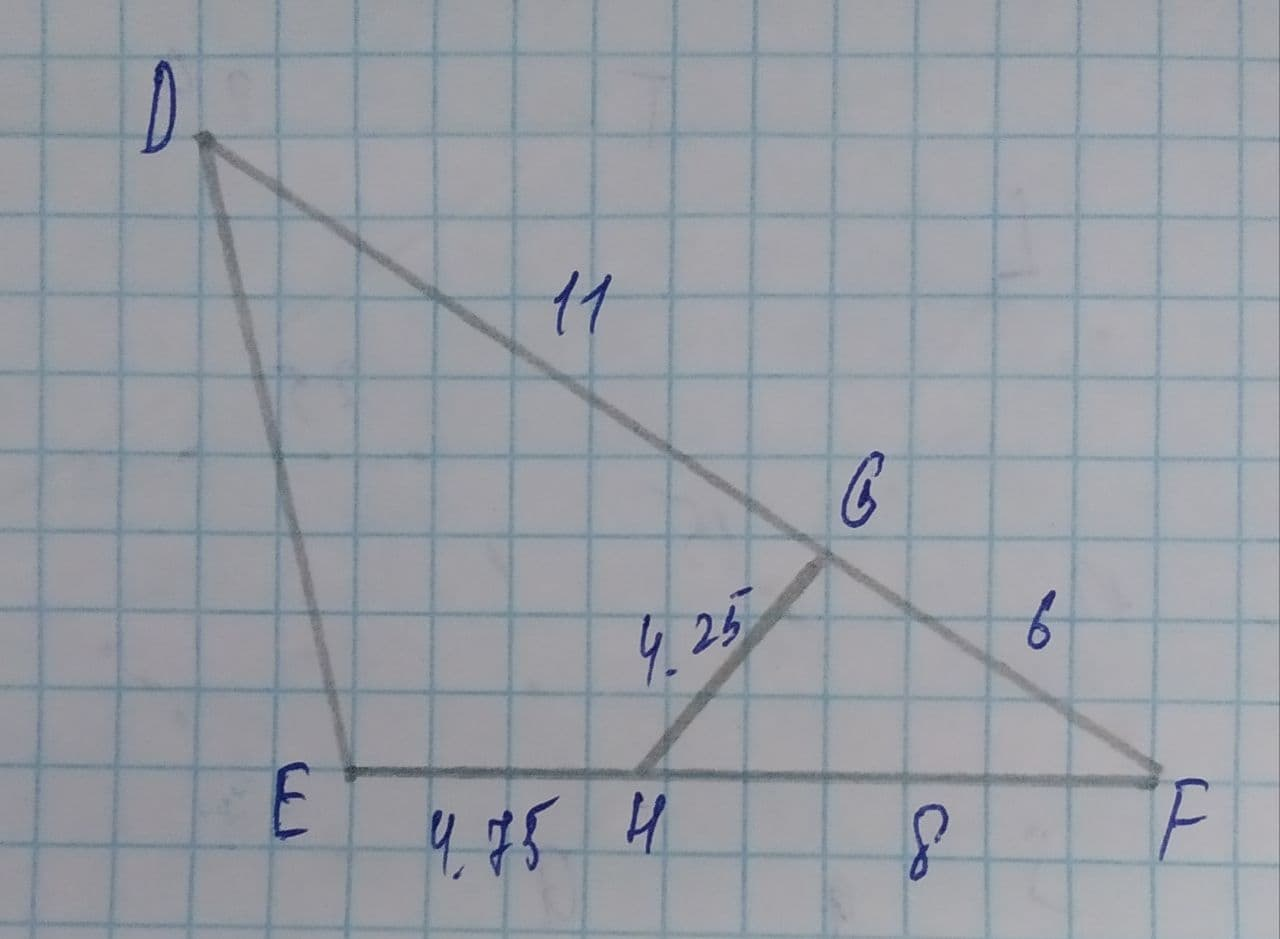# Given that \triangle FGH is similar to \triangle FED, Calculate DE to the hundedths place.Ayaana Buck 2021-08-11 Answered
Given that $$\displaystyle\triangle{F}{G}{H}$$ is similar to $$\displaystyle\triangle{F}{E}{D}$$, Calculate DE to the hundedths place.• Questions are typically answered in as fast as 30 minutes

### Plainmath recommends

• Get a detailed answer even on the hardest topics.
• Ask an expert for a step-by-step guidance to learn to do it yourself.delilnaT
Step 1
Triangle FGH is similar to triangle FED since, angle(F) is common to both the triangles,
and, angle(GHF) = angle(EDF)
and, remaining, angle(HGF) = angle(DEF)
Step 2
So, we have, by the triangle similarity,
$$\displaystyle{\frac{{{D}{E}}}{{{D}{F}}}}={\frac{{{H}{G}}}{{{H}{F}}}}$$
So, $$\displaystyle{\frac{{{D}{E}}}{{{\left({11}+{6}\right)}}}}={\frac{{{4.25}}}{{{8}}}}={0.53125}$$
So, $$\displaystyle{D}{E}={17}\times{0.53125}$$
So, DE=9.03125=9.03
So, DE=9.03